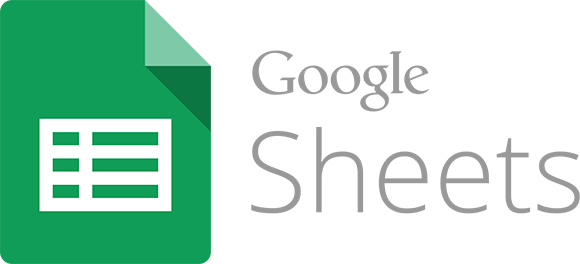This blog is a bit of a drawer of ICT stuff and today I want to talk about spreadsheets, which I haven’t made any article yet talking about formulas. And specifically, a feature that Google spreadsheets have. The IMPORTRANGE function.

For me, spreadsheets are one of the basic tools that a teacher must master. And not so much to make calculations of grades, but to order lists, group students, share data that is collected…

And in this last aspect, the IMPORTRANGE function is very useful and works very well. The idea is very simple and its name indicates it very well. It is used to import a range of cells from one calculation book to another book.

Until a year ago, the function was limited and often made mistakes. But it’s working well and fast these days.

The nomenclature is very simple:

The spreadsheet key is easy to locate. On Google Drive, each file has a code that identifies it. Simply open the document, in this case a workbook, and it is located at the URL address (after /d/ and before /edit…):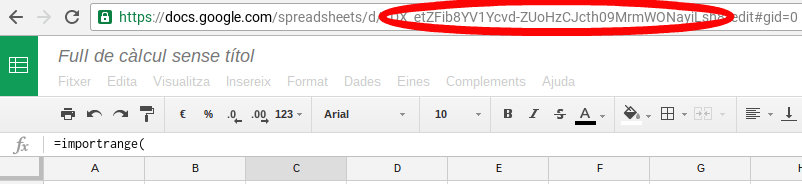An example would be the following
IMPORTRANGE(“1DX_etZFib8YV1Ycvd-ZUoHzCJcth09MrmWONayiLsh8”;“‘Sheet 1’!A1:A8”)

Our spreadsheet displays the values on Sheet 1, range A1: A8, of the book you have coded 1DX_etZFib8YV1Ycvd-ZUoHzCJcth09MrmWONayiLsh8.

With a great advantage, if these cells change in the original sheet, in the sheet where we are inserting the cells also change automatically.

In addition, as with all spreadsheet formulas, they can be combined. In this way, we could type the key of the sheet from where we want to import in a cell (for example B2) and in the formula, indicate the cell:

IMPORTRANGE(B2;“‘Sheet 1’!A1:A8”)

So we have more game to switch spreadsheets.

However, the first time we use this feature, it asks for permission. In fact, error #REF appears, and when you put the mouse over it, ask for permission: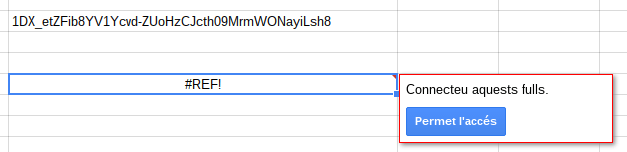If access is allowed, it is already easily imported.

What applications does this have in a facility? I basically use it in two areas.

With students: when students carry out data search tasks (surveys, measurements, etc.), each student can record the data in his or her own calculation book. As a teacher I have permission in all your books and I can create a new book that collects everyone’s data. I give permission to all students to read and quickly and easily all can see the data collected by their classmates, but they can not modify or delete them. Of course, I had to copy and paste all the keys from the students’ spreadsheets.

With teachers: in much the same way as above, you can share data that teachers collect.
In addition, we also use it extensively for project reporting. When working with projects, many aspects need to be evaluated. Some are evaluated with rubrics, others are corrected by the teachers, others leave the observation… In our case, each one ends up in a different calculation book. To make the final reports, we need to have it all in one book. And this is where the importrange function comes in. In a simple way, in a single book we collect the final evaluation of each of the aspects.

In fact, thinking for a while I can think of a few more examples where we use this feature. But let each one value what juice he or she can get: -)

ENLARGEMENT (June 2017)

I’ve been getting a lot of questions about how to combine the importrange function with other functions. Here is an example with the vlookup function.

In the following spreadsheet (origin), you can see a mini-database with products: A, B, C and D.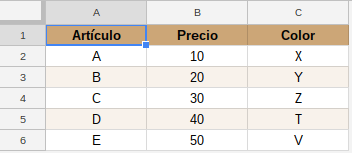Suppose that in another spreadsheet (destination) we want to capture some of these data, according to a cell. In other words, in another book we can indicate an article (example A) and automatically display the price and color.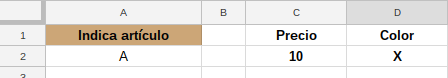What formula should we put in cell C2? What about the D2?

The formula in C2 would be as follows:

The formula in D2 would be as follows:

In other words, the vlookup function searches for the data from the importtrange function. But watch out, there’s a problem!

When using the importrange function alone, an error message appears due to lack of permissions and the possibility of connecting the sheets to fix it.When the importrange function is used in combination with other functions, the error appears, but not the possibility of connecting the leaves. I don’t know why, but I know the solution.

Simply use the importrange function alone first, give permission, and then use it in combination. In the example it gave, there would first of all, somewhere in the target book, have to indicate the following formula:

When the error appears, connect the leaves allowing access.

The leaves are now connected, so the formulas in cells C2 and D2 are already working correctly. The formula that we have put with importrange alone, if we want, we can erase it now.

1.Eric Desjardins says:

Hi, thanks for this very explanation of the use of Importrange function.

I have a question on this validation done on permission.

Is the permission is granted to access the source spreadsheet, though the sequence of action you propose, to the destination worksheet independent of the user that run the work sheet

or

does it have to be granted each and every time somebody nes is using the spreadsheet?¿?

Thanks again

Eric

1.Jaume Feliu says:

If a user authorizes, the sheets are connected for all users.
Moreover, if a user opens the target sheet, even if he does not have permissions on the source sheet, he will also see the imported data.

This site uses Akismet to reduce spam. Learn how your comment data is processed.